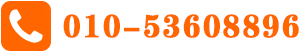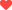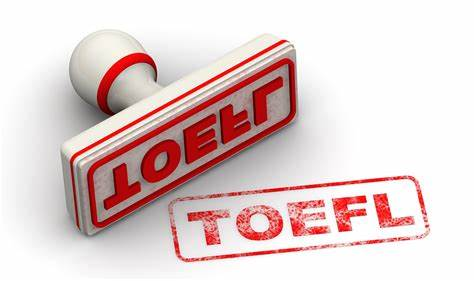# A-level基础数学知识串讲纯数

力学

统计

P1: Algebra Expression

Equations and inequities

Graphs and transformations

Straight line graphs

Trigonometric ratios

Differentiation

Integration

P2: Algebraic method

Coordinate Geometry

Exponents and Logarithm

The binomial expansion

Sequence and Series

Trigonometry identity and equations

Differentiation and Integration

P3: Algebraic Method

Functions and Graphs

Trigonometric functions

Exponentials and Logarithm

Differentiation

Integration

Numerical Method

P4: Proof

Partial Fraction

Coordinate Geometry

Binomial Expansion

Differentiation

Integration

Vectors

基础代数

方程与函数

线性代数

微积分喜欢 

## 最新文章 More## 相关推荐#### Alevel物理 | 动量问题一定要掌握的知识点

Alevel物理 | 动量问题一定要掌握的知识点.....

## 猜你喜欢 More